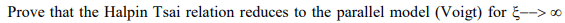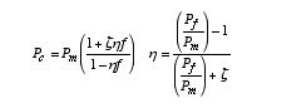# The Halpin Tsai relation to Voigt model

## Homework Statement## Homework Equations

Halpin Tsai equation:[/B]Voigt model:
P*=VfPf+VmPm

Vf= volume of fiber/ volume of composite
Vm= volume of matrix / volume of composite
ζ= estimated parameter
Pf, Pm= fiber and matrix properties
V_v=1-Vf-Vm

## The Attempt at a Solution

Since there is no V_v in the Halpin Tsai's equation, I am confused on how the Voigt Model obtained Vm from Halpin Tsai's equation

#### Attachments

•